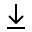MathBin Ellipse Member

An ellipse is a plane curve surrounding two focal points, such that for all points on the curve, the sum of the two distances to the focal points is a constant. It generalizes a circle, which is the special type of ellipse when both focal points are the same. The elongation of an ellipse is measured by its eccentricity e, a number ranging from e = 0 (the limiting case of a circle) to e = 1 (the limiting case of infinite elongation, no longer an ellipse but a parabola).

Here are the common conventions for the ellipse bins.

• The ContourPlot method plots the ellipse in blue.
• The center is the purple point.
• The two vertices are the blue points.
• The two foci are the black points.
• The two directrices are the dark green dashed lines.
• The two latus rectums are the brown dashed line segments.
• The two end points of the conjugate (minor) axis are the dark gray points.
• You can manually zoom out of the graph.
Bins in Two Dimensions